Ch. 1 - A Review of General ChemistryWorksheetSee all chapters
 Ch. 1 - A Review of General Chemistry 4hrs & 47mins 0% complete Worksheet Ch. 2 - Molecular Representations 1hr & 12mins 0% complete Worksheet Ch. 3 - Acids and Bases 2hrs & 45mins 0% complete Worksheet Ch. 4 - Alkanes and Cycloalkanes 4hrs & 18mins 0% complete Worksheet Ch. 5 - Chirality 3hrs & 33mins 0% complete Worksheet Ch. 6 - Thermodynamics and Kinetics 1hr & 19mins 0% complete Worksheet Ch. 7 - Substitution Reactions 1hr & 46mins 0% complete Worksheet Ch. 8 - Elimination Reactions 2hrs & 24mins 0% complete Worksheet Ch. 9 - Alkenes and Alkynes 2hrs & 10mins 0% complete Worksheet Ch. 10 - Addition Reactions 3hrs & 33mins 0% complete Worksheet Ch. 11 - Radical Reactions 1hr & 57mins 0% complete Worksheet Ch. 12 - Alcohols, Ethers, Epoxides and Thiols 2hrs & 34mins 0% complete Worksheet Ch. 13 - Alcohols and Carbonyl Compounds 2hrs & 14mins 0% complete Worksheet Ch. 14 - Synthetic Techniques 1hr & 28mins 0% complete Worksheet Ch. 15 - Analytical Techniques: IR, NMR, Mass Spect 7hrs & 18mins 0% complete Worksheet Ch. 16 - Conjugated Systems 5hrs & 49mins 0% complete Worksheet Ch. 17 - Aromaticity 2hrs & 24mins 0% complete Worksheet Ch. 18 - Reactions of Aromatics: EAS and Beyond 4hrs & 31mins 0% complete Worksheet Ch. 19 - Aldehydes and Ketones: Nucleophilic Addition 4hrs & 54mins 0% complete Worksheet Ch. 20 - Carboxylic Acid Derivatives: NAS 2hrs & 3mins 0% complete Worksheet Ch. 21 - Enolate Chemistry: Reactions at the Alpha-Carbon 1hr & 59mins 0% complete Worksheet Ch. 22 - Condensation Chemistry 2hrs & 13mins 0% complete Worksheet Ch. 23 - Amines 1hr & 43mins 0% complete Worksheet Ch. 24 - Carbohydrates 5hrs & 56mins 0% complete Worksheet Ch. 25 - Phenols 15mins 0% complete Worksheet Ch. 26 - Amino Acids, Peptides, and Proteins 2hrs & 54mins 0% complete Worksheet

# Degrees of Unsaturation

See all sections

Hydrocarbons fall into two categories: Saturated and Unsaturated- and both of these terms describe the number of hydrogens that carbon is bonded to.

###### Definition of Saturation

Concept #1: The difference between saturated and unsaturated molecules.

Transcript

Hey, guys, so now we're going to talk about a topic called index of hydrogen deficiency and this is a topic that usually isn't covered in the first chapter of most textbooks. So, you're probably thinking, “Johnny, why are you teaching this to me if I don't need to know it yet?” Well, because there's this other topic called constitutional isomers and that topic is much easier to understand if you already know the IHD rules.
So what I'm going to do here is I'm going to jump a little bit forward and teach you something that you don't need to know yet because it's going to make isomers so much easier to understand for this chapter. Let's go ahead and begin.
There's actually two different ways to compute the IHD and that is one structural and the other one is with molecular formula. What I'm going to do on this page is just show you the structural one which is actually the easier one and then we'll move on to molecular formula.
First of all, let's just talk about what is index of hydrogen deficiency. Well, what it has to do with is how unsaturated or saturated a molecule is. So what's that word saturated? Well, a saturated molecule is any molecule that has as many hydrogens as possible.
And there's this formula that we can use in all chemistry to figure out if it has as many hydrogens as possible. And that formula is – maybe you remember it from gen chem – it's 2n plus 2, where n is equal to the number of carbons.
So I have my 2n plus 2 rule. What that's going to do is it's going to predict for me how many hydrogens I need for my structure for it to be fully saturated, meaning that it has as many hydrogens as it can possibly hold. Any molecule that has less than that number, 2n plus 2, is going to be considered to be unsaturated.
These are words, saturated and unsaturated, they might sound familiar to you. That's because these are words we use actually in cooking. Saturated fat would actually be a fat – fats are these long carbon chains – it would be this fat that has as many hydrogens as possible. An unsaturated fat would be one that's missing a few hydrogens.
So you might be wondering, “Okay, Johnny, what makes carbons miss hydrogens? Why would you be missing some?” It turns out it only happens for two different reasons. I'm going to write this down. It happens for pi bonds. Remember that pi bonds just have to do with double bonds or triple bonds. They both have pi bonds. Then also, rings.
Rings are also going to take away some of the hydrogens from a molecule because what that means is that one of the ends is supposed to be CH3 and the other end is supposed to be CH3, but when you make a ring, they have to fuse together so that means that now you have to make them CH2's. What I'm trying to say is that a ring actually takes some hydrogens away from the carbon structure.

The equation 2n + 2 (where n = #carbons) predicts the number of hydrogens that an organic molecule should contain to be considered saturated.

• If the total number of hydrogens < 2n + 2, then the molecule is unsaturated.

Are the following molecules saturated?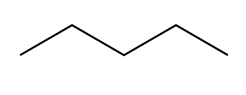Hint: You can figure this out without using the 2n + 2 rule.

Example #1: Is the following molecule saturated? Is it missing any hydrogens? How many if so?

Transcript

So if we use 2n plus 2, n in this case equals 5. So I'll do 2 times 5 plus 2 and that would mean that I need to have 12 hydrogens in order to be fully saturated. So then I would go ahead and ask myself does this have 12 hydrogens. And if you count what you would find is that would have 1, 2, 3, 4, 5, 6, 7, 8, 9 10, 11, 12. Wow! Look at that. I actually do have 12 hydrogens.
So that means that is this molecule saturated or unsaturated? This would be saturated.
That's the first thing, so now I just showed you a molecule that's saturated. Now another way that you could have know that this 2qs saturated, besides doing the formula, is you could have also asked yourself, okay, what makes things unsaturated? Well, pi bonds and rings. Did this chain have any pi bonds in it? No. It's all single bonds. Did it have any rings? No.
So also by looking at that, you could have said, “Oh, this is going to be a saturated molecule.” See, that's how this gets really easy.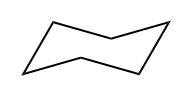Example #2: Is the following molecule saturated? Is it missing any hydrogens? How many if so?

Transcript

And it turns out that this one would be 2 times 6 plus 2, so we should have 14 hydrogens. But how many does it actually have? That's right, 12. I know you counted everyone, each one has 2. So this one actually has 12 hydrogens. Is it missing any hydrogens? Yes, it's actually missing two hydrogens.
Okay, does that make sense? So this would be considered unsaturated.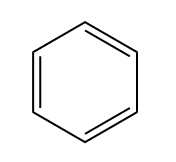Example #3: Is the following molecule saturated? Is it missing any hydrogens? How many if so?

Transcript

So this was also the same formula, 2 times 6 plus 2. It should have 14 H's. But how many does it actually have? Well if you think about bondline structure, there's only supposed to be 1 H in each of these positions, meaning that I only have 6 H's, meaning that I'm actually missing how many? I'm missing 8 H's. That's huge.
In the first one, I had all the H's possible. In the second one, I'm missing 2 and in the third one, I'm missing 8.

###### Predicting IHD Structurally

*IHD is also known as the Degree of Unsaturation.

Concept #2: What index of hydrogen deficiency is.

Transcript

So we know that we can use the words saturated and unsaturated to describe this, but is there a way to compare these guys? So is there a way to say, okay, which one is more unsaturated and which one is less unsaturated because they're both very different. The answer is yes. What we do is we use the IHD rules to really be very explicit about which one is the most unsaturated and which one is the least.
So the way that we do this is really easy. All we do is say that one IHD is the equivalent of missing two hydrogens. So if you're missing two hydrogens, that would be the equivalent of one index of hydrogen deficiency. That's just like the conversion.
The way that this works is that a ring or a double bond is going to count as one IHD, one IHD. And then a triple bond is going to count as two IHD because if you think about it a triple bond has two pi bonds in it so that would be two IHD.
And really that is it. Now instead of having to use that complicated formula – I mean the formula isn't hard, but it's just a little tedious sometimes. Imagine if you had like 11 carbons or something and you're having to maybe use more times tables then just your fingers or whatever. What I'm trying to say is that it can get tedious counting all those hydrogens out, so instead we use these rules.

Basically the IHD is an easy way for us to compare how unsaturated a molecule is compared to another.

• 1 IHD = Compound is missing 2 hydrogens.
• Rings/Double bonds = 1 IHD
• Triple Bonds = 2 IHD

What is the IHD of the following molecules?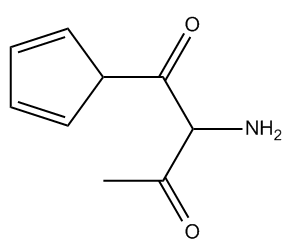Example #4: What is the IHD of the following molecule?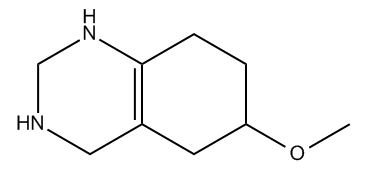Example #5: What is the IHD of the following molecule?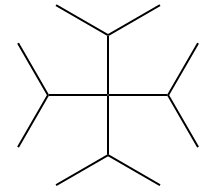Example #6: What is the IHD of the following molecule?

###### Predicting IHD using Molecular Formula

Unfortunately, the structure of a compound will not always be drawn for us. In that case, we use the following technique.

Concept #3: How to use IHD with molecular formula.

Transcript

When you're given a molecular formula to calculate IHD, you don't have the same clues that you had let's say if it was a structure. If you remember, we could use pi bonds or rings to figure out how many hydrogens were missing from a molecule. But when you're just given a molecular formula, let's say like, this example, C4H7Cl, I have no clue if there's rings there, if there's double bonds there. Really, all I have is the atoms.
So for this, we're going to have to use our own rule and our own formula because that's really the only way to solve it. Now your professor may have used a different rule in class, and in fact, most of the rules that I've seen are different from mine. The only reason I've settled on this one is because it worked for a lot of my students and they like it better. But if you have a rule that your professor taught you that you like better, please, by all means, use that one.
But this is the way it works, basically we need to calculate IHD and the way we do that is by calculating the theoretical H's. The way we do that is by using the formula 2n plus 2. So basically we calculate 2n plus 2. Then we subtract the actual number of hydrogens. But the thing is that for this we don't just use hydrogens, we also take into consideration other atoms that might act as hydrogen equivalents.
For example, when I'm calculating the number of hydrogens in a molecule, I don't just look at H, I also look at X's. Do you guys remember what X is? X would be a halogen and that counts as one hydrogen. For this formula, I count a halogen and a hydrogen exactly the same.
Then oxygen, what about if I see an oxygen in my compound? What do I could that as? Actually, I count that as zero. I ignore it. Whenever you see oxygen in this compound, just ignore it. That doesn't go into your equation.
Then how about nitrogen? Nitrogen is a special case. Nitrogen is actually going to count as negative one hydrogen for the overall compound. So that means that I'm not just counting H's, I'm also counting all halogens and all nitrogens and I'm ignoring the oxygens.
Finally, I get that number. That number is going to go here. This is going to be number of H's total. Then I'm going to take that number and I'm going to divide it all by 2, which is what I have here and that's going to be your IHD.
Now I know that sounds a little bit complicated, but once you get going with it, you can actually do it pretty fast.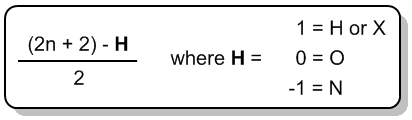*If your professor taught you a different method, that’s fine! Whatever helps you get the right answer.

Example #7: What is the IHD for C4H7Cl?

Example #8: What is the IHD for C6H7N?

Example #9: What is the IHD for C7H12O2?

Awesome job. Now we know how to easily calculate and compare the number of hydrogens that are missing for all types of compounds.